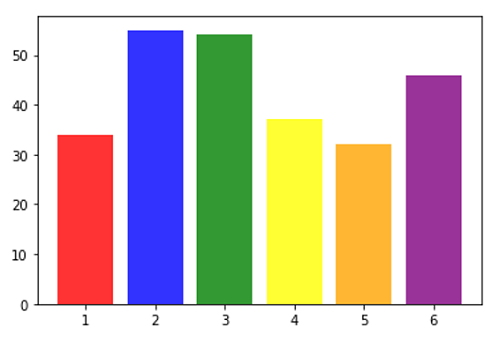# Python | Coloring Each Plot in Bar Plot

In this tutorial, we are going to learn how to color individual bars with different colors in a bar plot?
Submitted by Anuj Singh, on August 05, 2020

It is rarely used but, in some cases where we need to color different categories with different colors then we can use this property of matplotlib. The following example shows us how to implement colored bars in python using matplotlib.

Illustration:## Python code for coloring each plot in bar plot

```import matplotlib.pyplot as plt

x = [1,2,3,4,5,6]
y = [34, 55, 54, 37, 32, 46]

col = ['red', 'blue', 'green', 'yellow', 'orange', 'purple']

plt.figure()
plt.bar(x, y, color=col, alpha=0.8)
```

Output:

```Output is as figure
```

Languages: » C » C++ » C++ STL » Java » Data Structure » C#.Net » Android » Kotlin » SQL
Web Technologies: » PHP » Python » JavaScript » CSS » Ajax » Node.js » Web programming/HTML
Solved programs: » C » C++ » DS » Java » C#
Aptitude que. & ans.: » C » C++ » Java » DBMS
Interview que. & ans.: » C » Embedded C » Java » SEO » HR
CS Subjects: » CS Basics » O.S. » Networks » DBMS » Embedded Systems » Cloud Computing
» Machine learning » CS Organizations » Linux » DOS
More: » Articles » Puzzles » News/Updates